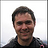Wrox Programmer Forums > C#Converting Easting/Northing to Latitude/Longitude
 |
 C# Programming questions specific to the Microsoft C# language. See also the forum Beginning Visual C# to discuss that specific Wrox book and code.
 Welcome to the p2p.wrox.com Forums. You are currently viewing the C# section of the Wrox Programmer to Programmer discussions. This is a community of software programmers and website developers including Wrox book authors and readers. New member registration was closed in 2019. New posts were shut off and the site was archived into this static format as of October 1, 2020. If you require technical support for a Wrox book please contact http://hub.wiley.com

 elwappoAuthorized User Join Date: Aug 2006 Posts: 41 Thanks: 1 Thanked 0 Times in 0 PostsConverting Easting/Northing to Latitude/Longitude

Does anybody have a pre-written class that can do this in C#? I need to be able to pass it an UK easting/northing (e.g. 262600, 191900) and return a Lat/Long (e.g. 51.3456, -3.17624).

Your help would be most appreciated.
Elwap.samjudsonFriend of Wrox Join Date: Aug 2007 Posts: 2,128 Thanks: 1 Thanked 189 Times in 188 PostsHere is your answer:

http://blogs.msdn.com/oldnewthing/ar...4/9068064.aspx

/- Sam Judson : Wrox Technical Editor -/

 elwappoAuthorized User Join Date: Aug 2006 Posts: 41 Thanks: 1 Thanked 0 Times in 0 PostsWhy thank you. Your answer was most informative. I'll be sure to seek advice here again.samjudsonFriend of Wrox Join Date: Aug 2007 Posts: 2,128 Thanks: 1 Thanked 189 Times in 188 Posts:)

It was meant to be a funnier way of saying, "I don't know"

I have written the code in PHP, but I don't know where I put it, so it's not that hard (provided you don't try to understand the math).

/- Sam Judson : Wrox Technical Editor -/

 elwappoAuthorized User Join Date: Aug 2006 Posts: 41 Thanks: 1 Thanked 0 Times in 0 PostsI certainly don't want to understand the math, as I've seen the formulas. That's why we have encapsulation :)

If you do find the code, I'd really appreciate it. Or if you can point me to a website which has a no frills way of describing how to implement the formula, I'd be most grateful. There must be a converter out there. Here's great online VB.NET to C# converter I use for code snippets all the time:

http://www.developerfusion.com/tools.../vb-to-csharp/samjudsonFriend of Wrox Join Date: Aug 2007 Posts: 2,128 Thanks: 1 Thanked 189 Times in 188 PostsCode:
```<?php

\$lon = 0;
\$lat = 0;

function convertXToLong(\$xCoord, \$yCoord)
{
global \$lat, \$lon;

\$E = \$xCoord;
\$N = \$yCoord;

\$a = 6377563.396;
\$b = 6356256.910;              // Airy 1830 major & minor semi-axes
\$F0 = 0.9996012717;                             // NatGrid scale factor on central meridian
\$lat0 = 49*pi()/180;
\$lon0 = -2*pi()/180;  // NatGrid true origin
\$N0 = -100000;
\$E0 = 400000;                     // northing & easting of true origin, metres

\$e2 = 1 - (\$b*\$b)/(\$a*\$a);                          // eccentricity squared
\$n = (\$a-\$b)/(\$a+\$b);
\$n2 = \$n*\$n;
\$n3 = \$n*\$n*\$n;

\$lat=\$lat0;
\$M=0;
do {
\$lat = (\$N-\$N0-\$M)/(\$a*\$F0) + \$lat;

\$Ma = (1 + \$n + (5/4)*\$n2 + (5/4)*\$n3) * (\$lat-\$lat0);
\$Mb = (3*\$n + 3*\$n*\$n + (21/8)*\$n3) * sin(\$lat-\$lat0) * cos(\$lat+\$lat0);
\$Mc = ((15/8)*\$n2 + (15/8)*\$n3) * sin(2*(\$lat-\$lat0)) * cos(2*(\$lat+\$lat0));
\$Md = (35/24)*\$n3 * sin(3*(\$lat-\$lat0)) * cos(3*(\$lat+\$lat0));
\$M = \$b * \$F0 * (\$Ma - \$Mb + \$Mc - \$Md);             // meridional arc

} while (\$N-\$N0-\$M >= 0.00001);  // ie until < 0.01mm

\$cosLat = cos(\$lat);
\$sinLat = sin(\$lat);
\$nu = \$a*\$F0/sqrt(1-\$e2*\$sinLat*\$sinLat);              // transverse radius of curvature
\$rho = \$a*\$F0*(1-\$e2)/pow(1-\$e2*\$sinLat*\$sinLat, 1.5);  // meridional radius of curvature
\$eta2 = \$nu/\$rho-1;

\$tanLat = tan(\$lat);
\$tan2lat = \$tanLat*\$tanLat;
\$tan4lat = \$tan2lat*\$tan2lat;
\$tan6lat = \$tan4lat*tan2lat;
\$secLat = 1/\$cosLat;
\$nu3 = \$nu*\$nu*\$nu;
\$nu5 = \$nu3*\$nu*\$nu;
\$nu7 = \$nu5*\$nu*\$nu;
\$VII = \$tanLat/(2*\$rho*\$nu);
\$VIII = \$tanLat/(24*\$rho*\$nu3)*(5+3*\$tan2lat+\$eta2-9*\$tan2lat*\$eta2);
\$IX = \$tanLat/(720*\$rho*\$nu5)*(61+90*\$tan2lat+45*\$tan4lat);
\$X = \$secLat/\$nu;
\$XI = \$secLat/(6*\$nu3)*(\$nu/\$rho+2*\$tan2lat);
\$XII = \$secLat/(120*\$nu5)*(5+28*\$tan2lat+24*\$tan4lat);
\$XIIA = \$secLat/(5040*\$nu7)*(61+662*\$tan2lat+1320*\$tan4lat+720*\$tan6lat);

\$dE = (\$E-\$E0);
\$dE2 = \$dE*\$dE;
\$dE3 = \$dE2*\$dE;
\$dE4 = \$dE2*\$dE2;
\$dE5 = \$dE3*\$dE2;
\$dE6 = \$dE4*\$dE2;
\$dE7 = \$dE5*\$dE2;
\$lat = \$lat - \$VII*\$dE2 + \$VIII*\$dE4 - \$IX*\$dE6;
\$lon = \$lon0 + \$X*\$dE - \$XI*\$dE3 + \$XII*\$dE5 - \$XIIA*\$dE7;

\$lat = \$lat*180/pi();
\$lon = \$lon*180/pi();
}
?>```
/- Sam Judson : Wrox Technical Editor -/

 elwappoAuthorized User Join Date: Aug 2006 Posts: 41 Thanks: 1 Thanked 0 Times in 0 PostsThanks, that worked a treat. :)Similar Threads Thread Thread Starter Forum Replies Last Post Plotting Latitude/longitude coordinates goodrags VB How-To 12 December 11th, 2007 01:31 PM converting dates dhoward VB.NET 2002/2003 Basics 6 August 2nd, 2007 08:42 AM Converting V.B.6 to V.B.2005 - Help ! liamfitz Intro Programming 1 October 31st, 2006 12:36 PM Converting cookies value. rupen Javascript How-To 6 June 8th, 2005 02:34 AM converting dates Bhavin Crystal Reports 1 October 13th, 2004 10:00 AMContact Us - Wrox - Privacy Statement - Top

Powered by vBulletin®
Copyright ©2000 - 2020, Jelsoft Enterprises Ltd.
Copyright (c) 2020 John Wiley & Sons, Inc.